10upon10.com

# Integrals NCERT Solutions

12-math-home

## NCERT Exercise 7.1

Find an anti derivative (or integral) of the following by the method of inspection:

Question: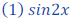Solution:

Given, sin2x

To find out the antiderivation we have to find out a function whose derivation is sin2x.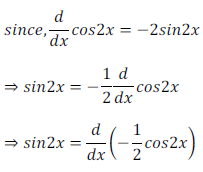Therefore, antiderivaion ofAlternate method:Therefore, antiderivaion ofQuestion: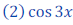Solution:

Given, cos3x

Since,Thus, antiderivative of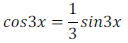Alternate method: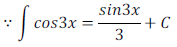Thus, antiderivative of12-math-home

Reference: